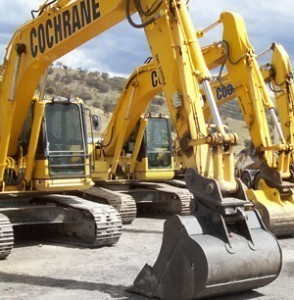# Excavator Sizes

Fixtures at most construction sites,excavators are manufactured according to precise specifications. The following is an overview of some of these vehicles.

### Excavator Dimensions: 307C, 307C SB & 308C CR (Caterpillar)

The model 307C has an operating weight of 6.49 metric tons and arm / stick measuring 5′ 6". The digging depth is 13′ 6" and the bucket capacity is 0.2 – 0.45 cubic yards (SAE heaped, min-max). The net hp is 54.

The 307C SB excavator weighs 7.66 metric tons and the arm is 5′ 6". The digging depth is 13′ 8" and the bucket capacity is 0.2 – 0.45. 54 is the net hp. The 308C CR has an operating weight of 8.04 and an arm length of 7′ 3". The maximum digging depth is 15′ 5" and the bucket capacity is 0.15 – 0.34.

### Excavator Dimensions: 311C Utility, 312C & 314C CR (Caterpillar)

The 311C Utility weighs 11.98 metric tons and has a 9′ 2" arm. The digging capacity is 18′ 4" and the bucket capacity is 0.39 – 0.97 cubic yards. The net hp is 79.

The 312C L excavator has an operating weight of 13.14 metric tons, an arm / stick measuring 9′ 10" and a digging depth of 19′ 10". The bucket capacity is 0.39 – 0.97 and the net hp is 90.

The 314C CR operating weight is 14.61 metric tons and the arm / stick is 9′ 10". The max dig depth is 19′ 6" and the bucket capacity is 0.39 – 0.97 cubic yards. The net hp is 90.

### Excavator Dimensions: Bobcat, Coyote, Gallmac & IHI

The Bobcat 442 has an operating weight of 7.502 metric tons and an arm stick measuring 6′ 7". The maximum dig depth is 13′ 11". The net hp is 72. The Coyote CE75 weighs 7.43 metric tons and has an arm length of 5′ 5.4". Dig depth is set at 13′ 2.3" and the net hp is 57.

The Gallmac CMW 70 excavator has an operating weight of 7 metric tons and a dig depth of 12′ 2". The net hp is 63. The IHI 70Z operating weight is 7.282 metric tons. The dig depth is 14′ 1" and the net hp is 57.

### Excavator Dimensions: Other Models

he Mustang ME 8002 has an operating weight of 7.9 metric tons. The dig depth is 14′ 5.2" and the operating weight of the Thomas T-75 is 7.45 metric tons. The max dig depth is 13′ 10.1" and the bucket capacity is 0.3259 cubic yards (SAE heaped). The net hp is 57.

The Yanmar B7SigmaC has an operating weight of 8.081 metric tons and the maximum digging depth is 14′ 3". The net hp is 58. The dig depth is 0.34 cubic yards. The net hp is 61. The Takeuchi TB175 has an operating weight of 7.815 metric tons. The arm length is 6′ 11" and the dig depth is 15′ 2". The bucket capacity is 0.27 – 0.34 and the net hp is 58.

The excavator dimensions cited here are just indicators of the specifications constructors and operators look at. Be sure to give the contractor specific details so the job gets done easily.